## Bipolar Coordinates

Bipolar coordinates are a 2-D system of coordinates. There are two commonly defined types of bipolar coordinates, the first of which is defined by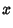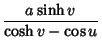(1)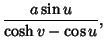(2)

where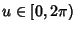,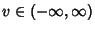. The following identities show that curves of constant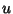and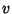are Circles in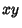-space.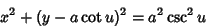(3)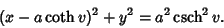(4)

The Scale Factors are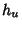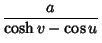(5)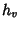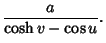(6)

The Laplacian is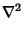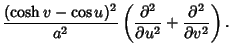(7)

Laplace's Equation is separable.

Two-center bipolar coordinates are two coordinates giving the distances from two fixed centers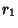and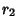, sometimes denoted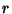and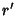. For two-center bipolar coordinates with centers at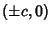,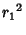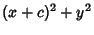(8)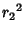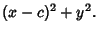(9)

Combining (8) and (9) gives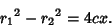(10)

Solving for Cartesian Coordinatesandgives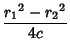(11)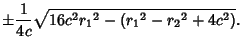(12)

Solving for Polar Coordinates gives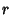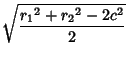(13)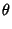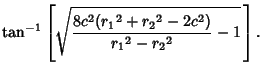(14)

References

Lockwood, E. H. Bipolar Coordinates.'' Ch. 25 in A Book of Curves. Cambridge, England: Cambridge University Press, pp. 186-190, 1967.